| my account | login-logout | resources | support | catalog | home | get webcard |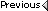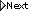» Online Classroom   » Inland and Coastal Navigation   » Public Discussion of Inland and Coastal Navigation   » Correcting for current

Author Topic: Correcting for current
 James Brown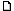posted November 03, 2005 10:56 AM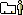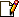I want to maintain a true course and speed over the ground 310 for 1.5 miles at 14 knots. There is a 2 knot current @ 270 degress. Using the Ferry angle calculation (current speed divided by ships speed then multiplied by 60 gives me 8.57. I add this number to the above course of 310, which would become 318. Is this correct?
 David Burchposted November 03, 2005 10:58 AMFirst, let me clarify some details related to this type of problem. Current drift and set of 2.0 at 270 means the current is flowing toward direction 270 T. That is the standard convention. Current directions given in true, as the direction they flow toward.Since current is true, then we assume the desired course is also true. ie we want to make good a course of 310 T in the presence of a current of 2 kts flowing in direction 210 T.The next point is more critical and not clear from the question. It has to do with the speed. You ask for a "speed over ground" of 14 kts, which is a problem we can solve, but the answer will not be unique... ie there are more than one set of courses to steer and speeds to turn that will end up with COG = 310 and SOG = 14. the answer to that question would be both a speed and a course.the implication of the question is you are looking for a course answer alone, and that would imply that your vessel speed was fixed at 14 kt. In this case, we could find the course to steer, turning 14 kts on the knotmeter, that will end up making good a course of 310 in the current given. The actual speed we make good on this course of 310, however, will not be 14.0.finding a course-speed combination that will end up with both a desired speed and a desired course is a different problem from the simpler one of knowing a fixed speed, what angle do i steer into the current to correct for a known set and drift.we can get the answer to that one either exactly by plotting or computation, or by the short cut presented in the text.The trick is based on the approximation that the amount we correct our course is the same as the amount we would get set if we do not correct it -- a very close, but not precise assumption.Then we further approximate that the set angle is drift/speed x 60° or x40° depending on the relative direction of current and desired course... as illustrated and explained in the caption to Figure 9-5 in the text. Using terminology from that picture, we have to decide if it is a beam current (use 60) or a bow or quarter current (use 40).So we point the boat in the direction we want to go (310) and ask where is the current (270) relative to the desired direction. In this case, a beam current would be coming from 90° to the right of 310, which is 310+90=400 (-360) = 040.... in other words, we want to go roughly NW, so a beam current would be from roughly NE... but our current is actually from 090 (flowing toward 270), so when we are pointed to 310, this current is closer to our quarter.This means we use the factor 40, and our actual SOG will be larger than 14. There is a component pushing us to the side and a component pushing us forward.the correction would be (2/14) x 40 = 5.7, or about 6°... and knowing this, we would point into the current by 6° to make good 310. Into the current is to the right, which means we add, and get 316° T as a course to steer.If you were to work out this problem precisely by computation or plotting, you would get 5.3° (ie C=315.3 T) and the resulting SOG would be 15.47 kts. In practice, this estimate is usually good enough since we do not know the set and drift precisely enough to justify a more sophisticated solution... in this case it was very close, but an error of even 5° would be usable.One last note: if you are preparing for a USCG license exam, then you will need to practice the precise plotting solution, since they could offer answers within 5°, and you would need to determine the final speed as well, which our trick does not do. From: Starpath, Seattle, WA

 All times are Pacific